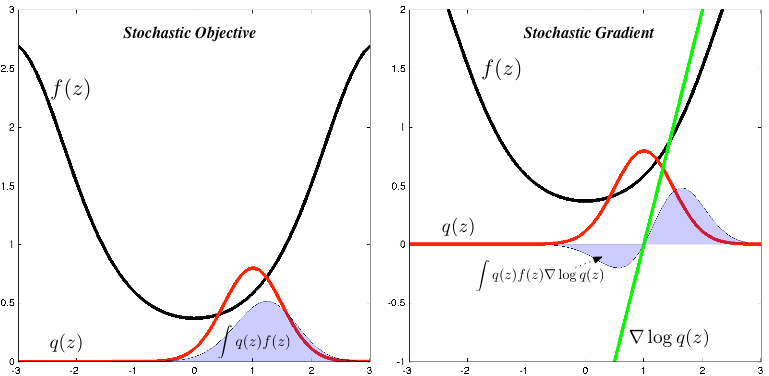# Machine Learning Trick of the Day (5): Log Derivative Trick

Machine learning involves manipulating probabilities. These probabilities are most often represented as normalised-probabilities or as log-probabilities. An ability to shrewdly alternate between these two representations is a vital step towards strengthening the probabilistic dexterity we need to solve modern machine learning problems. Today's trick, the log derivative trick, helps … Continue reading Machine Learning Trick of the Day (5): Log Derivative Trick